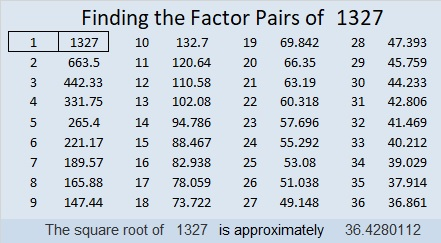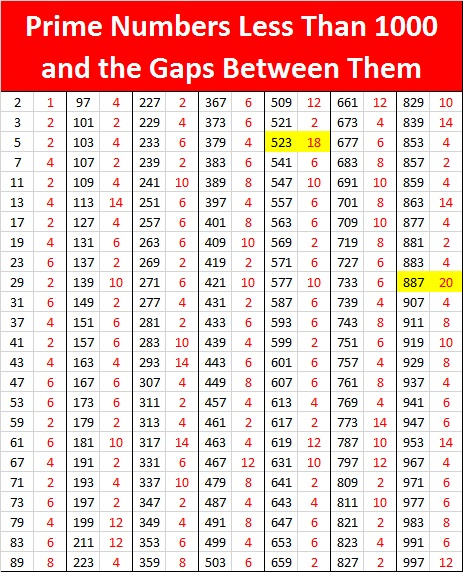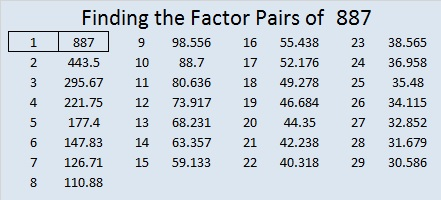1327 Record Breaking Prime Gap

Here is a table of prime numbers with the gaps between them and the next prime number. When a prime gap is larger than any previous prime gap, I’ve highlighted it in yellow. As you can see 1327 breaks the previous record of 22 held by 1129.That the next 33 numbers after 1327 are all composite is pretty remarkable. Even more remarkable is the fact that 1327 will hold the record for being the smallest prime number with the biggest prime gap until 9551 breaks it with a slightly larger prime gap of 36.

Here is some additional information about the number 1327:

• 1327 is a prime number.
• Prime factorization: 1327 is prime.
• The exponent of prime number 1327 is 1. Adding 1 to that exponent we get (1 + 1) = 2. Therefore 1327 has exactly 2 factors.
• Factors of 1327: 1, 1327
• Factor pairs: 1327 = 1 × 1327
• 1327 has no square factors that allow its square root to be simplified. √1327 ≈ 36.42801

How do we know that 1327 is a prime number? If 1327 were not a prime number, then it would be divisible by at least one prime number less than or equal to √1327 ≈ 36.4. Since 1327 cannot be divided evenly by 2, 3, 5, 7, 11, 13, 17, 19, 23, 29 or 31, we know that 1327 is a prime number.1129 is the Last Prime Number for a While!

The last prime number before 1129 was 1123.

The next prime number after 1129 will be 1151.

1151 – 1129 = 22

That’s the largest gap between primes so far! The previous record was 20. The chart below shows the gaps between all the prime numbers up to 1163.Here’s more about the number 1129:

• 1129 is a prime number.
• Prime factorization: 1129 is prime.
• The exponent of prime number 1129 is 1. Adding 1 to that exponent we get (1 + 1) = 2. Therefore 1129 has exactly 2 factors.
• Factors of 1129: 1, 1129
• Factor pairs: 1129 = 1 × 1129
• 1129 has no square factors that allow its square root to be simplified. √1129 ≈ 33.6006

How do we know that 1129 is a prime number? If 1129 were not a prime number, then it would be divisible by at least one prime number less than or equal to √1129 ≈ 33.6. Since 1129 cannot be divided evenly by 2, 3, 5, 7, 11, 13, 17, 19, 23, 29 or 31, we know that 1129 is a prime number.27² + 20²  = 1129

1029 is the hypotenuse of a Pythagorean triple:
329-1080-1129 calculated from 27² – 20², 2(27)(20), 27² + 20²

Here’s another way we know that 1129 is a prime number: Since its last two digits divided by 4 leave a remainder of 1, and 27² + 20² = 1129 with 26 and 21 having no common prime factors, 1129 will be prime unless it is divisible by a prime number Pythagorean triple hypotenuse less than or equal to √1129 ≈ 33.6. Since 1129 is not divisible by 5, 13, 17, or 29, we know that 1129 is a prime number.

1129 is also palindrome 1N1 in BASE 24 (N is 23 base 10)
because 24² + 23(24) + 1 = 1129

887 is the Last Prime Number for a While!

The last prime number before 887 was 883.

The next prime number won’t be until 907. Wow!907 – 887 = 20. That’s the largest gap between prime numbers so far!

887 is also a palindrome in a few other bases:

• 31313 BASE 4, because 3(4⁴) + 1(4³) + 3(4²) + 1(4) + 3(1) = 887
• 737 BASE 11, because 7(11²) + 3(11) + 7(1) = 887
• 313 BASE 17, because 3(17²) + 1(17) + 3(1) = 887

Those patterns of 3’s and 1’s for two different bases surprised me!

• 887 is a prime number.
• Prime factorization: 887 is prime.
• The exponent of prime number 887 is 1. Adding 1 to that exponent we get (1 + 1) = 2. Therefore 887 has exactly 2 factors.
• Factors of 887: 1, 887
• Factor pairs: 887 = 1 × 887
• 887 has no square factors that allow its square root to be simplified. √887 ≈ 29.782545

How do we know that 887 is a prime number? If 887 were not a prime number, then it would be divisible by at least one prime number less than or equal to √887 ≈ 29.8. Since 887 cannot be divided evenly by 2, 3, 5, 7, 11, 13, 17, 19, 23, or 29, we know that 887 is a prime number.